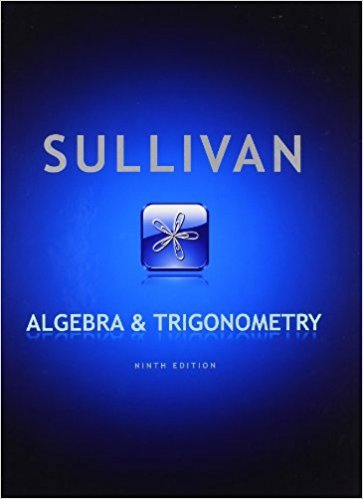×
×

# Solutions for Chapter 5.5: Algebra and Trigonometry 9th Edition## Full solutions for Algebra and Trigonometry | 9th Edition

ISBN: 9780321716569Solutions for Chapter 5.5

Solutions for Chapter 5.5
4 5 0 255 Reviews
14
5
##### ISBN: 9780321716569

This expansive textbook survival guide covers the following chapters and their solutions. Since 119 problems in chapter 5.5 have been answered, more than 61829 students have viewed full step-by-step solutions from this chapter. Algebra and Trigonometry was written by and is associated to the ISBN: 9780321716569. Chapter 5.5 includes 119 full step-by-step solutions. This textbook survival guide was created for the textbook: Algebra and Trigonometry, edition: 9.

Key Math Terms and definitions covered in this textbook
• Adjacency matrix of a graph.

Square matrix with aij = 1 when there is an edge from node i to node j; otherwise aij = O. A = AT when edges go both ways (undirected). Adjacency matrix of a graph. Square matrix with aij = 1 when there is an edge from node i to node j; otherwise aij = O. A = AT when edges go both ways (undirected).

• Affine transformation

Tv = Av + Vo = linear transformation plus shift.

• Basis for V.

Independent vectors VI, ... , v d whose linear combinations give each vector in V as v = CIVI + ... + CdVd. V has many bases, each basis gives unique c's. A vector space has many bases!

• Cofactor Cij.

Remove row i and column j; multiply the determinant by (-I)i + j •

• Complete solution x = x p + Xn to Ax = b.

(Particular x p) + (x n in nullspace).

• Factorization

A = L U. If elimination takes A to U without row exchanges, then the lower triangular L with multipliers eij (and eii = 1) brings U back to A.

• Free columns of A.

Columns without pivots; these are combinations of earlier columns.

• Full column rank r = n.

Independent columns, N(A) = {O}, no free variables.

• Hankel matrix H.

Constant along each antidiagonal; hij depends on i + j.

• Indefinite matrix.

A symmetric matrix with eigenvalues of both signs (+ and - ).

• Independent vectors VI, .. " vk.

No combination cl VI + ... + qVk = zero vector unless all ci = O. If the v's are the columns of A, the only solution to Ax = 0 is x = o.

• Iterative method.

A sequence of steps intended to approach the desired solution.

• Nilpotent matrix N.

Some power of N is the zero matrix, N k = o. The only eigenvalue is A = 0 (repeated n times). Examples: triangular matrices with zero diagonal.

• Nullspace N (A)

= All solutions to Ax = O. Dimension n - r = (# columns) - rank.

• Plane (or hyperplane) in Rn.

Vectors x with aT x = O. Plane is perpendicular to a =1= O.

• Projection p = a(aTblaTa) onto the line through a.

P = aaT laTa has rank l.

• Rayleigh quotient q (x) = X T Ax I x T x for symmetric A: Amin < q (x) < Amax.

Those extremes are reached at the eigenvectors x for Amin(A) and Amax(A).

• Standard basis for Rn.

Columns of n by n identity matrix (written i ,j ,k in R3).

• Vector space V.

Set of vectors such that all combinations cv + d w remain within V. Eight required rules are given in Section 3.1 for scalars c, d and vectors v, w.

• Wavelets Wjk(t).

Stretch and shift the time axis to create Wjk(t) = woo(2j t - k).

×